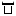shushanfx 2016-10-27 13:07:48

# javascript函数实现几分钟前，几小时前，几天前的代码片段

1. 与当前时间相差60s以内(不包括60s)，显示1分钟内；
2. 与当前时间相差60min以内(不包括60min)，显示XX分钟前；
3. 与当前时间相差24h以内(不包括24h)，显示XX小时前；
4. 与当前时间相差3d以内(不包括3d)，显示XX天前；
5. 发布时间为今年，显示MM-dd；
6. 发布时间不在今年， 显示yyyy-MM-dd;

```/**
* 获取指定时间的友好时间字符串。
* @param str 指定的时间字符串，如yyyy-MM-dd HH:mm:ss
* @param now 当前时间，允许时间戳，GMT时间，如果该参数为undefined，则使用浏览器时间。
*/
function getFriendlyTime(str, now){
var currentTime = new Date(now);
var arr = str.split(/\s+/gi);
var temp = 0, arr1, arr2, oldTime, delta;
var getIntValue = function(ss, defaultValue){
try{
return parseInt(ss, 10);
}catch (e){
return defaultValue;
}
};
var getWidthString = function(num){
return num < 10 ? ("0" + num) : num;
};
if(arr.length >= 2){
arr1 = arr.split(/[\/\-]/gi);
arr2 = arr.split(":");
oldTime = new Date();
oldTime.setYear(getIntValue(arr1, currentTime.getFullYear()));
oldTime.setMonth(getIntValue(arr1, currentTime.getMonth() + 1) - 1);
oldTime.setDate(getIntValue(arr1, currentTime.getDate()));

oldTime.setHours(getIntValue(arr2, currentTime.getHours()));
oldTime.setMinutes(getIntValue(arr2, currentTime.getMinutes()));
oldTime.setSeconds(getIntValue(arr2, currentTime.getSeconds()));

delta = currentTime.getTime() - oldTime.getTime();

if(delta <= 6000){
return "1分钟内";
}
else if(delta < 60 * 60 * 1000){
return Math.floor(delta / (60 * 1000)) + "分钟前";
}
else if(delta < 24 * 60 * 60 * 1000){
return Math.floor(delta / (60 * 60 * 1000)) + "小时前";
}
else if(delta < 3 * 24 * 60 * 60 * 1000){
return Math.floor(delta / (24 * 60 * 60 * 1000)) + "天前";
}
else if(currentTime.getFullYear() != oldTime.getFullYear()){
return [getWidthString(oldTime.getFullYear()), getWidthString(oldTime.getMonth() + 1), getWidthString(oldTime.getDate())].join("-")
}
else{
return [getWidthString(oldTime.getMonth() + 1), getWidthString(oldTime.getDate())].join("-");
}
}
return "";
}```
##### 猜你喜欢0个评论0个牛币最近下载最近浏览
lgdaijm  LV4 2020年5月17日
laoyaoshui  LV16 2018年12月11日扫描二维码关注最代码为好友